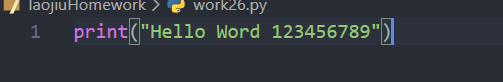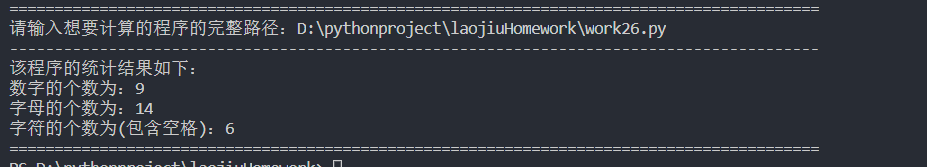• 判断有几个数字
千次阅读
2021-07-17 23:51:30

题目：

编写程序判断一个从键盘输入的字符串包含的字母、数字字符和其它字符的个数
程序分析：

遍历字符串，在遍历字符串时，判断该字符是什么类型的字符

代码：

letter = 0
num = 0
other = 0
str0 = input('请输入一段字符串：')
for i in str0:
if i.isalpha():
letter += 1
elif i.isdigit():
num += 1
else:
other += 1
print('字母个数为：', letter)
print('数字个数为：', num)
print('其他字符个数为：', other)

输出结果：

--------------------------------------------------------------------------------
请输入一段字符串：qaz123wsx456#!
字母个数为： 6
数字个数为： 6
其他字符个数为： 2
--------------------------------------------------------------------------------python
更多相关内容
• 字符串中可能：字母、数字和其它符号（比如标点符号） 方法一： charAt()方法、for循环和ASCII码 charAt(int index）返回下标位的字符 String a = "289r3f950tioperj02eko4tu90werjik294uewfji"; int ...

字符串中可能有：字母、数字和其它符号（比如标点符号）

方法一：

charAt()方法、for循环和ASCII码

charAt(int index）返回下标位的字符

       String a = "289r3f950tioperj02eko4tu90werjik294uewfji";
int number=0;//数字
int alphabet=0;//字母
int other=0;//其他
for(int i=0;i<a.length();i++){
if((a.charAt(i)>= 'a' && a.charAt(i)<= 'z') || ( a.charAt(i)>= 'A' && a.charAt(i) <= 'Z')){
alphabet++;
}else if((a.charAt(i)>= '0' && a.charAt(i)<= '9')){
number++;
}else{
other++;
}

}
System.out.println(number);//15
System.out.println(alphabet);//26

方法二：

toCharArray()方法、Character内置静态方法isLetter()和isDigit()和foreach函数

char[] chs = s1.toCharArray(); // 把字符串转换为字符数组

Character.isLetter(ch)//判断是不是数字

Character.isDigit(ch)//判断是不是字母

        String pr = "289r3f950tioperj02eko4tu90werjik294uewfji";
int numnum = 0;
int strnum = 0;
char [] chs = pr.toCharArray();
for(char ch:chs){
if (Character.isLetter(ch)){
strnum++;
}
else if(Character.isDigit(ch)){
numnum++;
}
else{
System.out.println("其他");
}
}
System.out.println("字母数量:"+strnum+"  数字数量："+numnum);

展开全文java 字符串
• 比较笨的方法，判断ASCII码： C# code? 1 2 3 4 5 6 7 8 9 10 11 12 13 14  string s = "abcd45612,asd";  int ...

比较笨的方法，判断ASCII码：

C# code?

 1 2 3 4 5 6 7 8 9 10 11 12 13 14             string s = "abcd45612,asd";             int characters = 0;             int numbers = 0;             int symbols = 0;             foreach (char c in s)             {                 if ((c >= 33 && c <= 47) || (c >= 58 && c <= 64) || (c >= 91 && c <= 96) || (c >= 123 && c <= 126))                     symbols++;                 if ((c >= 65 && c <= 90) || (c >= 97 && c <= 122))                     characters++;                 if (c >= 48 && c <= 57)                     numbers++;             }             Console.WriteLine("共有{0}个字母，{1}个数字，{2}个标点", characters, numbers, symbols);
展开全文• 输出: 程序中多少个字母, 多少个数字, 多少个符号 样例输入: print(“I don’t know!”); // 123456789 样例输出: 字母: 14 数字: 9 符号: 12 找到我想要计算的程序文件： 下面附上完整代码： numCount = 0 # ...

## 题目

某公司老板认为, 程序员按代码行算工资有漏洞
如果程序员不时的敲几个空行, 工资就上涨了不少
于是老板决定通过代码量来算工资
希望小伙伴写个程序判断一下, 一个程序有多少代码
输入: 本程序代码
输出: 程序中有多少个字母, 多少个数字, 多少个符号
样例输入:
print(“I don’t know!”);
// 123456789

样例输出:
字母: 14
数字: 9
符号: 12

找到我想要计算的程序文件：下面附上完整代码：

numCount = 0           # 数字计数器计数器  默认为0个
letterCount = 0        # 字母计数器  默认为0
charCount = 0          # 字符计数器  默认为0(字符包含空格)
print("="*90)
n = input("请输入想要计算的程序的完整路径：")

f = open(n, "r",encoding="utf-8")   # 只读打开文件，文本格式为utf-8
for i in text:                  # 对文件中的内容遍历
if i.isdigit():             # 如果遇到数字
numCount += 1
elif i.isalpha():           # 如果入到字母
letterCount += 1
else:                       # 如果是字符（包含空格）
charCount += 1
print("-"*90)
print("该程序的统计结果如下：")
print("数字的个数为：%d" %(numCount))
print("字母的个数为：%d" %(letterCount))
print("字符的个数为(包含空格)：%d" %(charCount))
print("="*90)
f.close()                       # 关闭文件


运行结果如下：展开全文python
•   在日常的编程中，经常会遇到求一组数据中是否相同数字的情况，这问题其实还是相对比较棘手的，我将用一道很经典的题来探讨这问题。 5位运动员参加了10米台跳水比赛，人让他们预测比赛结果 A选手说...
• 问题： Write a program that reads an integer, and determines and prints how many digits in the integer are 7s #include&lt;stdio.h&gt; #include&lt;stdlib.h&gt;......
• C语言，输入一段字符，判断有几个大写字母，几个小写字母，几个数字，几个空格… 写这个程序，我们可以借用指针，判断了一个字符后，指针向右移动一个单位，判断下一个，以此类推，一直到此字符被判断完。 代码如下 ...
• 今天遇到一简单的问题，判断整数具有即为有效数字，原来打算写一循环求余判断，最后想到可以把其转化成字符串，然后求取字符串的长度，就很好的解决了这问题。如：int a =123; int b=a.ToString().Length;...c#
• C++判断个数字位数 size_t countLenth(unsigned int i, size_t n = 1) { const size_t s = 10; if (i < s) { return n; } else { return countLenth(i / s, ++n); } }
• int a = 999999; System.out.println((a+"").length());java
• Java判断数字位数的方法总结发布于 2020-7-5|复制链接本文给大家整理了Java判断数字位数的两种常用方法，对此兴趣的可以跟着小妖一起学习下。普通方法：`javaimport java.util.Scanner;public class Digits {...
• [java]判断个数字中是否含有某个数字 例如判断个数字中是否含有2 代码： public class panduan { public static boolean test(int n) { while(n!=0) { if(n%10==2) { return true; } n/=10; } return ...算法 java
• js判断是否为数字方式很多： typeof、instanceof、Number.isNumber parseInt、parseFloat isNaN、isFinite 正则表达式 本片文章就介绍一下这些方式的区别和用法。 1. typeof、instanceof、Number.isInteger 使用...javascript
• ## 判断数字是几位数

千次阅读 2020-12-09 20:49:02
2.定义一方法,该方法的功能是计算该数字数字,并将位数返回 3.在main方法中打印该数字位数 4.演示格式如下: (1)演示一: 请输入一整数:1234 控制台输出:1234是4位数字 (2)演示二: 请输入一整数:-34567...
• 题目：输入数字范围，求这些数字中含有“2”，“0”，“1”，“9”的数，并求它们的和。 import java.util.Scanner; public class SpecialNum { public static void main(String[] args) { Scanner input = new ...
• " print("字母的数量:"+str(statistical(s))) print("数字的数量:" + str(statistical(s))) print("空格的数量:" + str(statistical(s))) print("其他的数量:" + str(statistical(s))) 输出结果: ...python
• 问题:用户输入数字判断数字位数。 代码1: #include <stdio.h> int main() { long long n; int count = 0; printf("输入一整数: "); scanf("%lld", &n); while(n != 0) { // n = n/10 n /= ...c语言 开发语言 后端
•c语言 开发语言
• 推荐手册：php完全自学手册is_numeric()函数用于检测变量是否为数字数字字符串。is_numeric()语法 (推荐学习：PHP视频教程)bool is_...返回值如果指定的变量是数字数字字符串则返回 TRUE，否则返回 FALSE。实例...
• python中如何判断一个数组中有几个不相同的数 输入 1 2 2 3 3 2 7 8 9 输出 6python
• ## java怎么判断是数字

千次阅读 2021-02-12 09:06:14
经常遇到要判断字符串中的字符是否是数字(0-9),判断字符串是不是数字，大家可能会用一些java自带的方法，也可能用其他怪异的招式，比如判断是不是整型数字，将字符串强制转换成整型，不是数字的就会抛出错误，...
• } } console.log(isOdd(3)) 解析：&会将数字转成2进制进行运算，0----000,1----001,2----010,3----011,4---100，以此类推。3&1等价于011&001，&是按位进行运算，两者都为1才是1，否则为0（运算规则：0&0=0; 0&1=0;...
• 判断数字位数 public class Test8{ public static void main (String []args){ weiShu(); } public static void weiShu(){ System.out.println(“打印参数n是位数”); java.util.Scanner s =new java....
• import java.util.Scanner;... *输入一个数字判断个数字代表星期 */ public class Test041508 { public static void main(String[] args) { //获取录入的一个数字 Scanner sc = new ...
• 判断字符串中出现数字的个数 a=input("请输入字符串") print("输入的字符串是",a) count=0 for i in a: if '0'<=i<='9': count+=1 print("字符串中出现数字的个数：{}".format(count))python 算法 字符串
• 用户输入数字判断数字位数。 代码如下： #include <stdio.h> void main(){ long n,j; int count=0; printf("请输入数字\n"); scanf("%d",&n); j=n; while(n!=0){ n =n/10; count++; ...
• 一、封装代码： public static boolean rangeInDefined(int current, int min, int max) { return Math.max(min, current) == Math.min(current, max); } 二、测试类： public static void main(String[] args) ...
• 数字华容道NxN数字随机排列的阵列解的充要条件是：N为奇数，总逆序数为偶数，N为偶数，总逆序数为奇数。即满足此条件，数字随机排列阵列，能通过移动数字方格，使阵列的数字按顺序排列。python
• 请输出任意一重复的数字，即判断数字是否重复即可。例：输入数组：{ 1, 6, 5, 3, 12, 2, 3, 2, 0, 1, 7, 4, 5 }打印出1、5、3、2任意一即可。方法如下三种：一、用一额外的数组记录重复情况，因为元素的值不...
• ## C++判断数字位数

千次阅读 2021-06-11 20:31:21
#include using namespace std; int main() { int n; int p=0; int a=0; int j=0; cout请输入你要求的位数"<>n; while(n!=0) { j=n%10; a+=j; n/=10; p++; }; cout这是"...